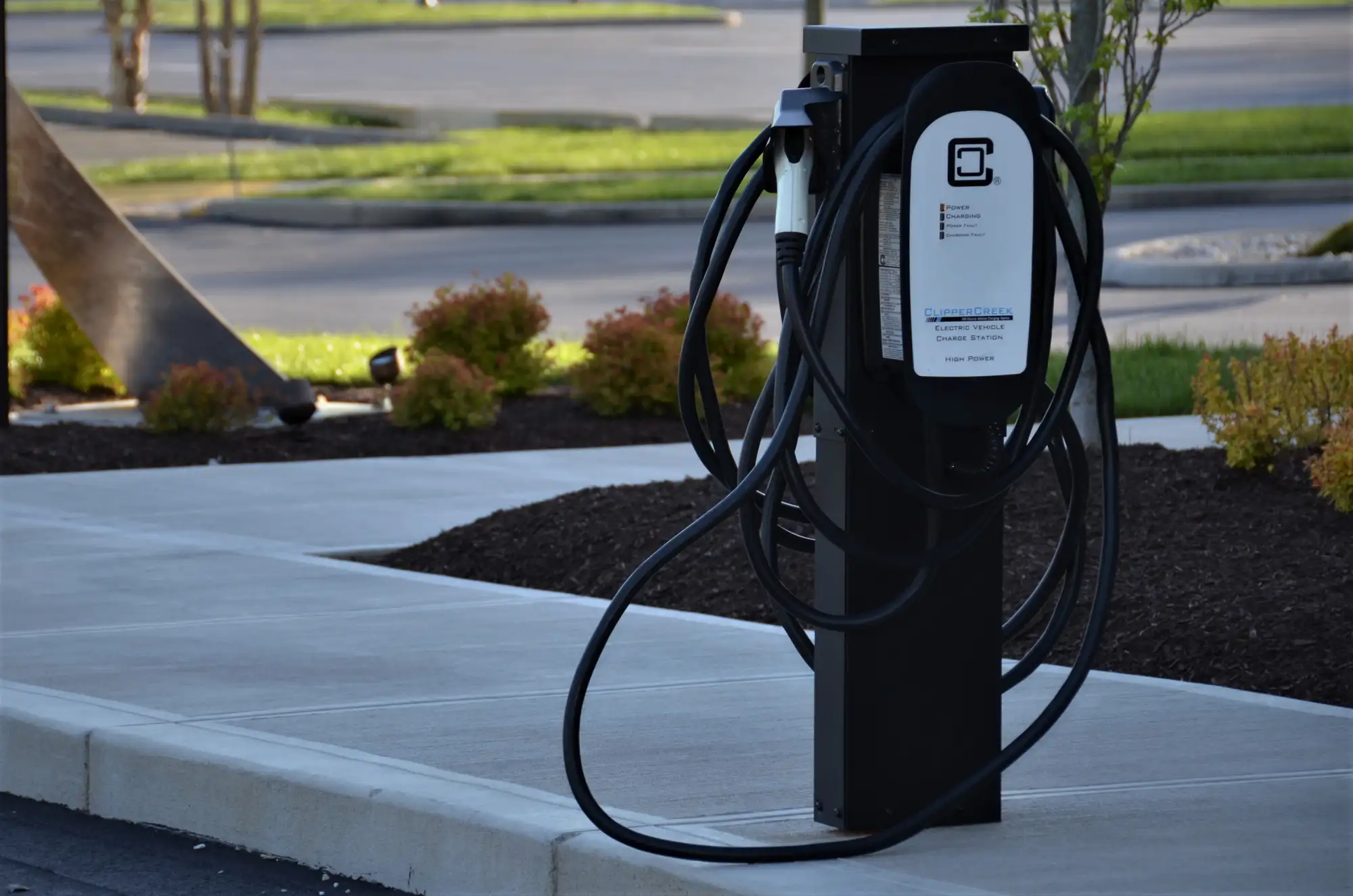# Understanding Electric Vehicle (EV) Fuel Equivalents## Introduction

Are you finding it difficult to compare the energy efficiency of EVs with traditional gasoline cars? People often struggle to understand how electricity relates to petrol in terms of metrics — that is because it's measured in a completely different way for EVs than for ICEs.

Electric car fuel equivalents are then an important tool when we talk about how energy-smart EVs are compared to regular cars. In simpler terms, they give us an easy way to match up how much energy EVs use against the usual "miles per gallon" (MPG) for our American friends, or “Liter per 100km” (l/100km) for us in the EU, that we think about with gas cars. This idea helps us see how different types of car technology stack up against each other and makes choosing an EV less confusing for anyone thinking about buying one.

Here's what fuel equivalent means: it's all about the energy. One litre of gas holds about 8.9 kilowatt-hours (kWh) of energy. So, when we talk about the EV fuel equivalent, often shown as kilometres per litre equivalent (Km/Le), we're saying how many kilometres an EV can go on the same amount of energy that's in a single litre of gas (that's the 8.9 kWh we just talked about). The key point here is that the higher the Km/Le, the better the energy efficiency of the EV.

## How Can EV Fuel Equivalents Help?

1. Universal Comparison: EV fuel equivalents provide a familiar metric (Km/L), easing understanding for consumers.
2. Efficiency Highlight: These equivalents underscore the superior energy efficiency of electric vehicles.
3. Sustainable Choices: By making energy efficiency clear, fuel equivalents can encourage more eco-friendly vehicle choices.
4. Fuel Cost Analysis: Consumers can estimate potential fuel cost savings using the fuel equivalent metric.
5. Policy Making Aid: Fuel equivalents can guide energy and transportation policies by providing a benchmark for EV efficiency.

## Tesla’s EV fuel Equivalent Table

Now, let's use an example to illustrate this concept more clearly. We'll look at Tesla’s fuel equivalent table as provided on their European Union Energy Label support page. The table displays the energy consumption of various Tesla models in kWh/100km, an EU standard for indicating the energy efficiency of an EV. For our example, let's pick the Tesla Model 3 Long Range, which uses 15 kWh/100km.

To make sense of this in terms of fuel equivalents let’s make an example: we have 15,5 kWh/100 kilometres. Then we figure out how many of these 'EV kilometres' can go into the energy that's in a litre of gas (8.76 kWh). This gives us a fuel equivalent of roughly 1,76 litres/100km. In comparison to a regular petrol car (BMW 3 series, with 6,5 litres/100km) it is almost 4 times less.

## Comparison of Tesla and BMW 3 series

To calculate the cost of driving a Tesla for 100km, we would need to know its efficiency in kWh/100km and the cost of electricity per kWh. As for a regular petrol car like an Audi or BMW, we would need to know its fuel consumption in liters/100km and the cost of petrol per liter.

### Tesla Model 3 Long Range:

Tesla Model 3 Long Range has an efficiency of approximately 15 kWh/100km. Assuming the cost of electricity is about 0.42 Euros per kWh (average price in Germany as of 2023), the cost for 100km would be:

15.5 kWh/100km * 0.42 Euro/kWh = 6.5 Euros

### Regular Petrol Car - BMW 3 series:

Let's take a BMW 3 series as an example, which might have a fuel consumption of approximately 6,5 litres/100km (medium). If the cost of petrol is about 1.89 Euros per litre (the price in June 2023), the cost for 100km would be:

6.5 liters/100km * 1.83 Euros/liter = 11.9 Euros

In this example, the Tesla would be almost twice less expensive to drive the same distance as the BMW.

## Conclusion

EV fuel equivalents are really helpful in understanding and comparing how energy-smart different vehicles are. By changing the energy use of an EV into a measurement that's easier for everyone to understand, folks looking to buy can really see how energy-efficient electric cars are. But remember, while these numbers are useful, they're just one piece of the puzzle. When thinking about how a car performs overall, how cost-effective it is, and what it means for the environment, there's more to consider. But EVs are proving to beat petrol-powered cars more and more on all criteria now.

## FAQ

### Q1: What is EV fuel equivalent?

A: EV fuel equivalent is a metric that enables the comparison of energy usage between electric vehicles and conventional gasoline-powered vehicles. It calculates how many kilometres an electric vehicle can travel using the same amount of energy contained in one litre of gasoline (approximately 8.9 kWh).

### Q2: How can I compare the cost of driving a Tesla versus a conventional petrol car?

A: For a Tesla, multiply its energy efficiency in kWh/100km by the cost of electricity per kWh. For a petrol car, multiply its fuel consumption in liters/100km by the cost of petrol per liter. For example, a Tesla Model 3 Long Range in Germany costs approximately 6.5 Euros to drive 100km, while a BMW 3 series costs approximately 11.895 Euros for the same distance.

### Q3: Are EV fuel equivalents the only measure of a car's efficiency?

A: No, while EV fuel equivalents are a useful tool for comparison, they are just one aspect of a car's overall efficiency. Other factors to consider include the vehicle's performance, cost-effectiveness, and environmental impact.

## Got sparks? We're amped to help!

Give us a call or drop us a line an we’ll get back to you ASAP.View FAQSend a message

Contact formSend a message

Contact form